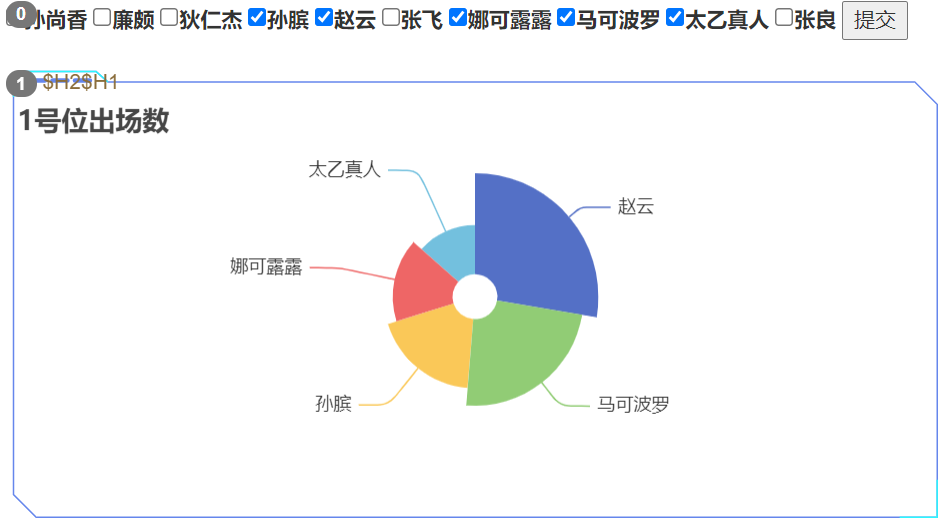### 应用场景 #

• Smartchart对于单项筛选有通用的组件， 可以直接配置即可
• 但对于个性化的筛选，需要你进行一些简单的定制化，
• 以下就针对多项筛选联动的需求来介绍如何定制化你的筛选联动效果
• 通过此例,你可以了解如何自定义任意的联动效果### 样列参考 #

• 新建一个图形组件
• 在新的数据集中编写查询
``````select distinct H1 as heroname  from smartdemo2 limit 10
``````
• 编辑此数据集的图形，可复制以下代码
``````//生成html组件
let dataset=__dataset__;
let table = '';
for (let i=1;i<dataset.length;i++){
table = `\${table}<label><input name="select__name__" type="checkbox" value="\${dataset[i]}" />\${dataset[i]}</label> `;
}
table = table + "<button id='id_select__name__'>提交</button>";
dom__name__.innerHTML=table;

//button 的点击响应
\$('#id_select__name__').click(()=>{
let res = [];
//获取所有选项并放入res列表中
\$("input[name='select__name__']:checked").each(function(i){
res.push("'" + \$(this).val() + "'");
});
let H1 = res.toString(); //拼接成参数
ds_setParam('H1',H1);  //参数赋值
ds_refresh(1);  //刷新1号图
}
)
``````
• 在你要联动刷新的目标数据集中增加代码
``````select H1 as heroname, sum(qty) as 出场数 from smartdemo2
where 1=1
/* and H1 in (\$H1) */
group by H1
order by sum(qty) desc
``````

TIPS